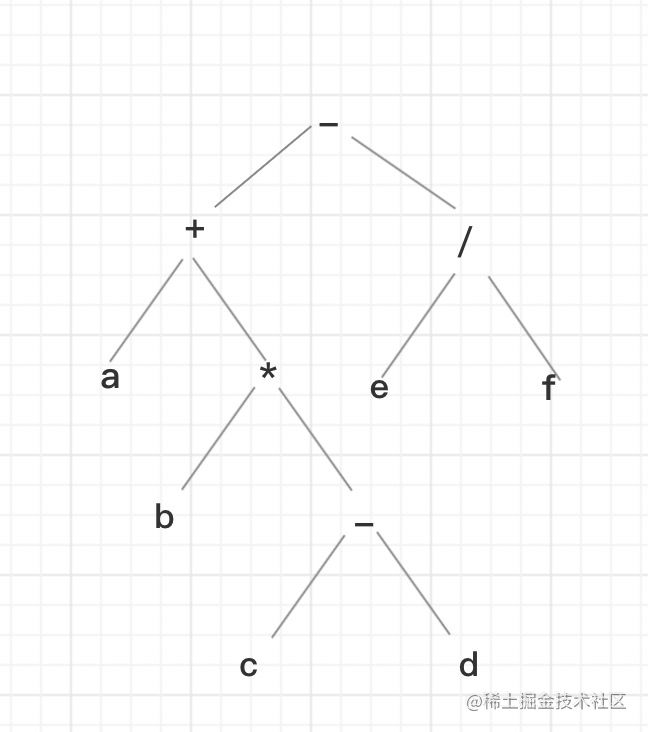﻿ 利用JS实现二叉树遍历算法实例代码_javascript技巧_脚本之家
javascript技巧利用JS实现二叉树遍历算法实例代码

前言

• 结点：每个元素称为结点
• 树根：根节点
• 度：一个结点含有的子结点的个数称为该结点的度
• 叶子节点：度为0的节点

一、二叉树

1.1、遍历二叉树

• 前序：先访问根，然后访问左子树，最后访问右子树，DLR。
• 中序：先访问左子树，然后访问根，最后访问右子树，LDR。
• 后序：先后访问左子树，然后访问右子树，最后访问根，LRD。
• 广度：按层的顺序一层一层遍历。• 前序：-+a*b-cd/ef
• 中序：a+b*c-d-e/f
• 后序：abcd-*+ef/-
• 广度：-+/a*efb-cd

1.2、用js表示二叉树

var tree = {
value: '-',
left: {
value: '+',
left: {
value: 'a'
},
right: {
value: '*',
left: {
value: 'b'
},
right: {
value: '-',
left: {
value: 'c'
},
right: {
value: 'd'
}
}
}
},
right: {
value: '/',
left: {
value: 'e'
},
right: {
value: 'd'
}
}
}

1.3、前序遍历算法

function preOrder(treeNode) {
if(treeNode) {
console.log(treeNode.value); // 打印出来代表访问这个节点
preOrder(treeNode.left);
preOrder(treeNode.right);
}
}

function preOrder(treeNode) {
if(treeNode) {
var stack = [treeNode]; //将二叉树压入栈
while (stack.length !== 0) {
treeNode = stack.pop(); // 取栈顶
document.getElementById('text').appendChild(document.createTextNode(treeNode.value)); // 访问节点
if(treeNode.right) stack.push(treeNode.right); // 把右子树入栈
if(treeNode.left) stack.push(treeNode.left); // 把左子树入栈
}
}
}

1.4、中序遍历算法

function InOrder(treeNode) {
if(treeNode) {
InOrder(treeNode.left);
document.getElementById('text').appendChild(document.createTextNode(treeNode.value));
InOrder(treeNode.right);
}
}

function InOrder(node) {
if(node) {
var stack = [];             // 建空栈
//如果栈不为空或结点不为空，则循环遍历
while (stack.length !== 0 || node) {
if (node) {               //如果结点不为空
stack.push(node);     //将结点压入栈
node = node.left;     //将左子树作为当前结点
} else {                  //左子树为空，即没有左子树的情况
node = stack.pop();   //将结点取出来
document.getElementById('text').appendChild(document.createTextNode(node.value));
node = node.right;    //将右结点作为当前结点
}
}
}
}

1.5、后序遍历算法

function postOrder(node) {
if (node) { //判断二叉树是否为空
postOrder(node.left); //递归遍历左子树
postOrder(node.right); //递归遍历右子树
document.getElementById('text').appendChild(document.createTextNode(node.value));
}
}

function postOrder(node) {
if (node) { //判断二叉树是否为空
var stack = [node]; //将二叉树压入栈
var tmp = null; //定义缓存变量
while (stack.length !== 0) { //如果栈不为空，则循环遍历
tmp = stack[stack.length - 1]; //将栈顶的值保存在tmp中
if (tmp.left && node !== tmp.left && node !== tmp.right) { //如果存在左子树,node !== tmp.left && node !== tmp.righ 是为了避免重复将左右子树入栈
stack.push(tmp.left);   //将左子树结点压入栈
} else if (tmp.right && node !== tmp.right) { //如果结点存在右子树
stack.push(tmp.right);  //将右子树压入栈中
} else {
document.getElementById('text').appendChild(document.createTextNode(stack.pop().value));
node = tmp;
}
}
}
}

stack       tmp         node        打印

1.6、按层遍历算法

if (node) {                             //判断二叉树是否为空
var que = [node];                   //将二叉树放入队列
while (que.length !== 0) {          //判断队列是否为空
node = que.shift();             //从队列中取出一个结点
document.getElementById('text').appendChild(document.createTextNode(node.value));   //将取出结点的值保存到数组
if (node.left) que.push(node.left);   //如果存在左子树，将左子树放入队列
if (node.right) que.push(node.right); //如果存在右子树，将右子树放入队列
}
}
}

二、算法题

1.1、二叉树的最大深度

3
/ \
9  20
/  \
15   7

const tree = {
value: 3,
left: {
value: 9
},
right: {
value: 20,
left: { value: 15 },
right: { value: 9 }
}
}

var maxDepth = function(root) {
if (!root) {
return 0;
}
const leftDeep = maxDepth(root.left) + 1;
const rightDeep = maxDepth(root.right) + 1;
return Math.max(leftDeep, rightDeep);
};
/*
maxDepth(root) = maxDepth(root.left) + 1  = 2
maxDepth(root.left) = maxDepth(root.left.left) + 1 = 1
maxDepth(root.left.left) = 0;

maxDepth(root) = maxDepth(root.right) + 1 = 3
maxDepth(root.right) = maxDepth(root.right.right) + 1 = 2
maxDepth(root.right.right) = maxDepth(root.right.right.right) + 1 = 1
maxDepth(root.right.right.right) = 0
*/

1.2、二叉树的所有路径

3
/ \
9  20
/  \
15   7

var binaryTreePaths = function(root) {
if (!root) return [];
const res = [];
function dfs(curNode, curPath) {
if(!curNode.left && !curNode.right) {
res.push(curPath);
}
if(curNode.left) {
dfs(curNode.left, `\${curPath}->\${curNode.left.value}`)
}
if(curNode.right) {
dfs(curNode.right, `\${curPath}->\${curNode.right.value}`)
}
}
dfs(root, `\${root.value}`);
return res;
};